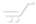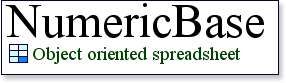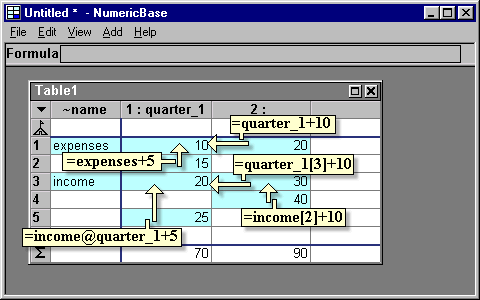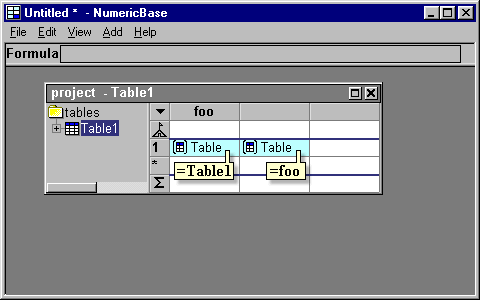# More about referring to other cells

More about referring to other cells

Referring to cells at the same table
You can refer to cells at the same table using column names, row names, column number, row number and combinations of these, as shown in the following table and screenshot.

 Formula Meaning Example (see screenshot below) column_name Cell at the same row and a column named column_name. =quarter_1+10 row_name Cell at the same column and a row named row_name =expenses+5 column_name[row_number] Cell at a field named column_name and a row numbered column_number. =quarter_1+10 row_name[column_number] Cell at a row named row_name and a column numbered column_number. =income+10 row_name@column_name Cell at a row named row_name and a column named column_name =income@quorter_1+5
Table: referring to cell at the same tableScreenshot: referring to cell at the same table

Referring to elements from other tables
You can refer to elements from other tables. To do that, you need to construct a formula that has two parts. The first part generates a reference to a table and the second part extract information from that reference. The table reference can be to any table in the solution, including the current table, and instantiated table.

For example, the formula =Table1.amount refers to the amount column in the table named Table1. The formula part Table1 gets a table reference, and the formula part .amount extracts information from that reference.

Getting a table reference.
One way to generate a table reference is to use the table name of the referenced table. Another way is to reference a cell that contain a table reference. The following screenshot illustrate the two ways: The cell containing the formula =Table1 uses the first alternative, and cell containing the formula =foo uses the second. Both generate the exact same table reference - to Table1, which is incidentally the same table that contain the formulas.Screenshot: getting a table reference

Referring to values from a table reference.
You can refer to values from a table reference by using the column name, column number, row name, and row number. The following table is showing the different ways of doing this, and the screenshot below show the table, named Table1, that used in the examples.

 Formula and example Meaning. Formula:table_ref.column_name Example:Table1.quarter_1 evaluates to 100 Cell at the column named column_name and the base row in the table referenced by table_ref. Formula: table_ref. row_name Example:Table1.expences Cell at the row named row_name and the base column in the table referenced by table_ref (planned). Formula: table_ref.column_name[row_number] Example:Table1.quarter_1 evaluates to 3 Cell at the column named column_name and the row numbered row_number in the table referenced by table_ref. Formula:table_ref.row_name@column_nameExample: Table1.expenses@quarter_1 Cell at the column named column_name and the row named row_name in the table referenced by table_ref (planned). Formula:table_ref [row_number]Example:Table1 evaluates to 10 Cell at the row numbered row_number and the base column of the table referenced by table_ref.
Table: referring to values from other tablesScreenshot: the table used in the examples above

Getting a row reference
If the referenced table does not have a base column, then the formulas
1. table_ref.row_name
2. table_ref [row_number]
Mentioned in the above table, returns a references to the appropriate rows, instead of the value at the base column. Another way to get a row reference is to you the "find" function.

Extracting values from a row reference
You can extract values from a row reference. The different ways to do that are described in the following table. In this table, row_ref can be any formula that generates a row reference.

 Formula Meaning row_ref[column_number] Cell at a column numbered column_number and the row referred to by row_ref row_ref.column_name Cell at a column named column_name and the row referred to by ref
Table: extracting values from row reference

Resolving ambiguities.
Sometimes, a name in a formula has more than one corresponding elements in the solution. For example, a table can have both a column and a row named amount (not recommended but allowed).

The name is resolved according to following priories listed from high to low:
1. Column name in the same table.
2. Row name in the same table
3. Table name in the solution.
4. Build-in function with zero arguments
If the name refers to an element at another object, as in: Table.name_at_difrrent_object, then the table name and build-in name are not used (priorities 3 and 4).